## 1 Introduction

As the performance of embedded processors rapidly increases, there is a growing trend toward integrating multiple real-time applications into a partitioned scheduling system in avionics development. The ARINC 653 standard  prescribes a robust temporal partitioning mechanism for integrated modular avionics (IMA) systems, where a global scheduler assigns a fraction of processor time to a temporally isolated partition that contains a set of concurrent tasks. A local scheduler of the partition manages the included tasks. The application of partitioned scheduling is effectively able to prevent failure propagation among partitions. However, it raises the question of how to allocate processor time to partitions in an optimal manner while guaranteeing their time requirements.

In ARINC 653, the time allocation for partitions is executed cyclically according to a static schedule. A schedulable system requires sufficient time allocation for all partitions. The time requirement of a partition is described as a tuple of periodic scheduling parameters $$\langle \textit{period},\textit{budget}\rangle$$, which can be used for generating the static schedule . Given the set of specific real-time applications in the system, these parameters determine not only the schedulability of the system but also its processor occupancy. In this paper, the question of resource allocation is interpreted as the optimization of ARINC-653 partition scheduling parameters of a schedulable system. The goal is to minimize the processor occupancy of the system, thus making it possible to accommodate more additional workload of applications .

The nature of ARINC-653 partition scheduling is a complex nonlinear non-convex parameter optimization problem . So far, most investigations [13, 20, 21, 29, 33] have been confined to analytical methods, whose rigorous mathematical models build on the worst-case assumptions of a simplified system. In more complex real-time applications, more expressive model-checking (MC) approaches [8,9,10,11, 22, 32] are extensively being developed to incorporate a great variety of behavioral features including concrete task actions, dependency and communications. They are based on various formal models such as preemptive Time Petri Nets (pTPN), Linear Hybrid Automata (LHA), and Timed Automata (TA). For each promising scheduling scheme, its schedulability can be verified or falsified automatically via state space exploration of the system model.

However, to identify a globally optimal scheduling configuration, the entire combinatorial parameter space must be explored thoroughly. Each of these combinations leads to a single model-checking operation which is in itself a PSPACE-complete problem. Therefore, we use Evolutionary Algorithm (EA) as a heuristic optimization method, thereby avoiding the brute-force search of parameter space.

The model-based methods are also confronted with the state space explosion problem, which makes the exact model checking practically infeasible. There have been several promising techniques that attempt to mitigate the state space explosion of classical MC. Statistical Model Checking (SMC)  is a simulation-based method that runs and monitors a number of simulation processes, providing the statistical results of verification with a certain degree of confidence. However, SMC cannot provide any guarantee of schedulability but quick falsification owing to its nature of statistical testing. By contrast, compositional approaches  decompose the system into components, check each component separately by classical MC and conclude system properties at a global level, but might offer conservative results due to abstraction of the components. Therefore, it is reasonable to combine the global SMC and compositional MC techniques. Nevertheless, we found no studies that applied such a combination to the optimization of ARINC-653 partition scheduling.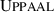is a model-checking toolbox for modeling and verifying real-time systems described as extended TA, which is expressive enough to cover features of an IMA system. There are several branches in thefamily. The classicalandSMC  provide the implementation of symbolic MC and SMC, respectively. In the previous work , we have integrated the global SMC and compositional MC into a-based schedulability analysis of IMA systems.

In this paper, we propose a model-based optimization method of ARINC-653 partition scheduling for IMA systems. The core idea is to extend theTA model of the system with a parameter sweep application that searches for the optimal schedulable solutions with respect to minimum processor occupancy. Our main contributions include:

• A model-based optimization method that addresses the optimal time allocation of partitioned scheduling systems by performing a heuristic search of the objective parameter space of theTA model.

• A-based modeling and analysis technique that supports parameter sweep by quickly falsifying non-schedulable solutions and evaluating schedulable ones. An IMA system is modeled as TA models inand its schedulability constraints are verified automatically via the integrated method of global SMC and compositional MC analysis.

• A generator of ARINC-653 partition schedules that connects the parameter optimizer and theTA models of an IMA system to enable the automatic design of IMA partition scheduling.

• An evolutionary algorithm that combines refined genetic search operators and the adaptation of evolution strategies, thereby accelerating the process of finding optimal solutions and meanwhile reducing the risk of premature convergence.

The rest of the paper is organized as follows. Section 2 gives the definition of the optimization problem. Section 3 provides a background of the schedulability analysis. Section 4 introduces the parameter optimization method and briefly presents its constituent components. We detail the evolutionary algorithm EA4HS in Sect. 5. The experiments on sample systems are shown in Sect. 6. Section 7 gives the related work and Sect. 8 finally concludes.

## 2 Optimization problem description

In this section, we first outline an IMA partitioned scheduling system, and then give the definition of its parameter optimization problem.

### 2.1 System model

We focus on a two-level partitioned scheduling system where partitions are scheduled by a Time Division Multiplexing (TDM) global scheduler and each partition also has a local scheduler based on preemptive Fixed Priority (FP) policy to manage the partition’s internal tasks.

The system consists of a set of temporal partitions $$\varOmega =\{\mathcal {P}_i|i=1,2,\dots ,n\}$$ running on a single processor. The TDM global scheduler executes time allocation for partitions according to a static schedule $$\mathcal {S}$$ cyclically and repeats $$\mathcal {S}$$ every major time frame M . The partition schedule $$\mathcal {S}$$ is comprised of a set of partition time windows: $$\mathcal {S}=\{W_t|t=1,2,\dots ,w\}$$. $$W_t$$ is a time slot $$\langle P_t,o_t,d_t\rangle$$ belonging to a partition $$P_t\in \varOmega$$, where $$o_t$$ and $$d_t$$ denote the offset from the start of M and expected duration respectively. The w time slots are non-overlapping, satisfying that $$0\le o_1<o_1+d_1<o_2<o_2+d_2<\dots<o_w<o_w+d_w\le M$$. Partitions are activated only during their partition time windows within M.

Each partition $$\mathcal {P}_i$$ accommodates a set of tasks $$\varGamma _i=\{\tau ^i_j|j=1,2,\dots ,m_i\}$$ which are scheduled by the local scheduler of $$\mathcal {P}_i$$ in accordance with the preemptive FP policy and executed only when $$\mathcal {P}_i$$ is activated. A task $$\tau$$ is represented by the tuple $$\langle I,T,O,J,D,R,L\rangle$$ where I is initial offset, T is release interval, O is offset, J is jitter, $$D\le T$$ is deadline, R denotes task priority, and L describes the behavior of $$\tau$$ as a sequential list. Each element of L is an abstract instruction $$\langle {{Cmd}, {Res}, T_{BCET}, T_{WCET}}\rangle$$. $$Cmd$$ is an operation code in the command set $$\{{Compute},$$ $$Lock,$$ $$Unlock,$$ $$Delay,$$ $$Send,$$ $$Receive,$$ $$End \}$$. $$Res$$ is an identifier encoding one of the resources such as processor time, locks, and messages. $${\textit{T}_{\textit{BCET}}}$$ and $${\textit{T}_{\textit{WCET}}}$$ are execution time in the best case and the worst case respectively. In the command set, $$Compute$$ denotes a general computation step, $$Lock$$ and $$Unlock$$ handle locks, $$Delay$$ allows the task to stop running for a certain time, $$Send$$ and $$Receive$$ are used for inter-partition communications, and $$End$$ is the symbol of job termination.

### 2.2 Schedulability condition

The schedulability of a partitioned scheduling system can also be divided into conditions at the global and the local level. Figure 1 shows this hierarchical scheduling architecture.

At the global level, the schedulability is that the time supply of the global scheduler satisfies the time requirement of each partition. The partition schedule $$\mathcal {S}$$ defines the time supply for partitions in the system. According to the ARINC 653 standard, the time requirement of a partition $$\mathcal {P}_i$$ can be described as a tuple of periodic scheduling parameters $$\langle p_i,b_i\rangle$$ where $$p_i$$ is a partition period and $$b_i$$ is the budget within $$p_i$$. Thus, the schedulability condition denotes that the budget $$b_i$$ can be guaranteed by the partition schedule $$\mathcal {S}$$ during each period $$p_i$$. Compared with the variable-length partition schedule, we are more interested in handling the concise parameter tuple $$\langle p_i,b_i\rangle$$ that is used as an input in determining the partition time windows of $$\mathcal {P}_i$$ .

The schedulability at the local level requires all tasks to meet their deadlines. The tuple of scheduling parameters $$\langle p_i,b_i\rangle$$ indicates the total periodic time requirement of tasks in $$\mathcal {P}_i$$. We define two types of tasks:

• A periodic task has the kth release time $$t_k\in [I+kT+O,I+kT+O+J]$$ where $$k\in \mathbb {N}$$ and T denotes a fixed period. A periodic task meets its deadline iff the task can finish its kth job before the instant $$(I+kT+D)$$ for any $$k\in \mathbb {N}$$.

• A sporadic task characterized by a minimum separation T between consecutive jobs releases its $$(k+1)$$th job at $$t_{k+1}\in [t_k+T,+\infty )$$, and its first release is at $$t_0\in [I,+\infty )$$. A sporadic task complies with its deadline iff its kth job can be completed before $$(t_k+D)$$ for any $$k\in \mathbb {N}$$.

In addition, the ARINC-653 standard allows tasks to perform two types of communication between them: intra- and inter-partition communication. The type of a communication operation of a task depends on whether the communicating tasks are located in the same partition. The behavior of resource sharing or message communication incurs the task-blocking overheads that could affect the schedulability of partitions at the local level. Hence, our model-based method also needs to describe the concrete task behavior including the (intra- and inter-partition) communication precisely inmodels.

### 2.3 Optimization problem

Consider the aforementioned partitioned scheduling system. Given a set of partitions $$\varOmega =\{\mathcal {P}_i|i=1,2,\dots ,n\}$$ and their respective task sets $$\{\varGamma _i\}$$, the optimization problem is to find a 2n-dimensional vector $$\mathbf {x}=(x_1,x_2,\dots ,x_{2n})\in \mathbb {R}^{2n}_+$$ where the parameter tuple $$\langle p_i,b_i\rangle$$ of $$\mathcal {P}_i$$ corresponds to the elements $$x_{2i-1}=p_i$$ and $$x_{2i}=b_i$$, such that the system minimizes the processor occupancy U while guaranteeing the schedulability at both the global and local level.

Suppose each release of partitions needs a context switch. The processor occupancy is defined as

\begin{aligned} U=\sum _{i=1}^n\frac{c_i\cdot v+b_i}{p_i}, \end{aligned}
(1)

where $$c_i$$ is the average number of context switching for $$\mathcal {P}_i$$ during each partition period $$p_i$$, and v is the context-switch overhead.

Minimizing the processor occupancy of a partitioned scheduling system makes it possible to accommodate more additional workload of applications. Similar definitions of the processor occupancy function have been proposed and applied in previous papers [13, 33], where it was called “processor utilization” or “system utilization.” We found these names counter-intuitive, because we normally chase a higher “utilization” but it should be minimized in this problem. Thus we renamed it processor occupancy in this paper. Equivalently, we also define the remaining processor utilization $$U_r=1-U$$ and find the maximum $$U_r$$ instead.

## 3 Background of schedulability analysis

In this section, we formulate the schedulability constraints of the optimization problem on the basis of the modeling formalism of. The behavior of the partitioned scheduling system presented in Sect. 2.1 is further modeled as a set oftemplates. A template is a generalized object of TA in. The automaton structure of a template consists of locations and edges. A template may also have local variables and functions. The templates can be instantiated as a network of TA model instances $$\mathcal {M}$$ that describe a complete system. For any scheduling parameter vector $$\mathbf {x}$$, the schedulability of its system model is verified or falsified according to the procedure in Fig. 2, where the right is the flowchart of our model-based analysis and the left dashed-line box contains the data objects of each process.

First, an ARINC-653 partition schedule $$\mathcal {S}$$ is generated automatically from the input parameter vector $$\mathbf {x}$$ via an partition scheduling algorithm, which guarantees $$\mathcal {S}$$ satisfies the time requirement of $$\mathbf {x}$$, i.e., schedulability at the global level. We refer to $$\mathbf {x}$$ as a valid parameter combination if a partition schedule can be generated from $$\mathbf {x}$$, then the schedulability analysis will proceed with the following costly steps. Otherwise, it will conclude with the invalidity of $$\mathbf {x}$$. A partition scheduling algorithm is presented in Sect. 4.2.

Second, the schedulability constraints of the optimization problem are expressed and fast falsified as queries of hypothesis testing inSMC. We add a boolean array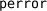with the initial value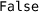to TA templates for this purpose. Once the schedulability of partition $$\mathcal {P}_i$$ is violated, the related model will assign the valueto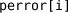immediately. Thus, the schedulability constraints for $$\mathcal {P}_i$$ are replaced with the following query $$\rho _i$$: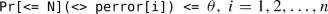(2)

where N is the time bound on the simulations and $$\theta$$ is a very low probability.SMC is invoked to estimate whether the system model $$\mathcal {M}$$ satisfies the conjunction of n queries statistically:

\begin{aligned} \mathcal {M}\models \rho _1\wedge \rho _2\wedge \dots \wedge \rho _n \end{aligned}
(3)

SinceSMC approximates the answer using simulation-based algorithms, we can falsify any nonschedulable solution rapidly but identify schedulable ones only with high probability ($$1-\theta$$). Note that the probability distributions used in such models affect the probabilities of events in the overall model. In our case, this is not important as we do not evaluate the probability of the events, but only search for a single trace violating the schedulability. Therefore, all schedulable results of SMC testing should be validated by classical MC to confirm the schedulability of the corresponding system.

Finally, in order to alleviate the state-space explosion problem of classical MC, we apply our compositional method presented in  to schedulability validation, which is comprised of the following four steps:

1. 1.

Decomposition The system model $$\mathcal {M}$$ is first decomposed into a set of communicating partitions models $$\mathcal {P}_i,\ i=1,2,\dots ,n$$. The schedulability property is also divided into n TCTL (Timed Computation Tree Logic) safety properties $$\varphi _i$$: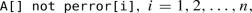(4)

each of which belongs to one partition.

2. 2.

Construction of message interfaces We define a message interface $$\mathcal {A}_i$$ as the assumption of the communication environment for each partition $$\mathcal {P}_i$$. $$\mathcal {A}_i$$ contains a set of TA models that mimic the requisite message-sending behavior of the other partitions.

3. 3.

Model checking We check each partition model $$\mathcal {P}_i$$ including its environment assumption $$\mathcal {A}_i$$ individually by verifying the local properties $$\varphi _i$$:

\begin{aligned} \mathcal {P}_i\Vert \mathcal {A}_i\models \varphi _i,\ i=1,2,\dots ,n \end{aligned}
(5)

where the operator $$\Vert$$ denotes composition of two TA models.

4. 4.

Deduction According to the assume-guarantee paradigm, we assemble the n local results together to derive conclusions about the schedulability of an entire system $$\mathcal {M}$$.

The optimization method proposed in the next section builds on the above analysis approach, which guarantees the schedulability constraints in search of the optimal solutions.

## 4 Parameter optimization method

The parameter optimization method presented in this section belongs to a class of random search methods. The optimizer searches for the (nearly) optimal schedulable parameters with respect to minimum processor occupancy U. Each search point in the considered parameter space can be converted into a promising ARINC-653 partition schedule. We finally give atemplate framework that describes an IMA partitioned scheduling system as a network of TA models.

### 4.1 Parameter sweep optimizer

The optimizer is structured as a Parameter Sweep Application (PSA) that comprises a set of independent “experiments”, each of which is performed by a PSA task with a different set of parameters . These PSA tasks tentatively explore the parameter space of $$\langle p_i,b_i\rangle ^n$$ to find promising search points.

For any search point $$\mathbf {x}$$, the optimizer creates a PSA task that carries out the following procedure depicted in Fig.3:

(1) A search algorithm first offers a promising parameter vector $$\mathbf {x}$$ to the PSA task. (2) An ARINC-653 partition schedule is then generated from the parameter setting of $$\mathbf {x}$$. (3) The PSA task instantiates themodeling framework by assigning the partition schedule to the TA models and (4) subsequently invokesSMC to execute a fast global schedulability test. (5) If the TA model goes through the SMC test, it should be validated byclassic via compositional analysis. (6) The schedulability constraints and processor occupancy are evaluated by the objective function. (7) The search algorithm receives feedback on the evaluation of $$\mathbf {x}$$ to update its candidate solutions and exploration direction. (8) Finally, this PSA task finishes its experiment and waits for the next call from the optimizer. The optimizer will continue the parameter sweep, based upon the results of previous experiments, until the optimization criteria are reached. The best scheduling parameter vector of $$\mathbf {x}$$ and its partition schedule will be output at the end of the parameter sweep.

Each component of the parameter sweep optimizer copes with a specific issue of the optimization problem.

A search algorithm guides the parameter sweep to select search points until an acceptable solution is found. We consider that exhaustive search is mostly infeasible and derivative information also unavailable for complex systems, thus employing an evolutionary algorithm to perform a heuristic search of the parameter space. Since there are no communications or data dependencies among PSA tasks, we adopt parallel search policies that distribute PSA tasks over several computing nodes so as to speed up the parameter sweep. Section 5 details the design of this evolutionary algorithm.

An ARINC-653 schedule generator converts the parameter vector $$\mathbf {x}$$ into an ARINC-653 static partition schedule by using an offline scheduling algorithm, which can make all scheduling decisions prior to run-time. This generator connects the parameter sweep optimizer and theTA models of an IMA system to enable the automatic design of ARINC-653 partition scheduling. Section 4.2 gives an implementation of the generator based on the preemptive FP scheduling policy.

Atemplate framework describes a partitioned scheduling system as a network of TA models. Sincesupports arrays and user-defined types, the ARINC-653 partition schedule is encoded into a structure array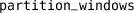where each element corresponds to a partition time window. The global scheduler modeled as a TA template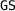executes partition scheduling according to the array records. When instantiating the templates, a PSA task should assign the array of its partition schedule to a copy of themodel file. Thetemplates are presented in Sect. 4.3.

The schedulability constraints of the optimization problem are expressed as three properties: (1) validity of $$\mathbf {x}$$, (2) hypotheses of the SMC testing, and (3) TCTL safety properties in the MC compositional analysis. For any $$\mathbf {x}$$, the schedulability of its corresponding system is verified or falsified in the form of these properties according to the procedure in Sect. 3. The results of this schedulability analysis are transferred from the ARINC-653 schedule generator orto the objective function in the optimizer.

The objective function of the optimization problem provides a quality evaluation for any parameter vector $$\mathbf {x}$$. Since the processor occupancy U of Eq. (1) is only valid for schedulable parameter vectors, we define the objective of the evolutionary search as a fitness function, which evaluates the remaining processor utilization $$U_r$$ of any $$\mathbf {x}$$ on the basis of schedulability constraints. The evaluation of $$\mathbf {x}$$ is to update the state and search direction of the evolutionary algorithm. We give the definition of this fitness function in Sect. 5.2.

### 4.2 Generation of ARINC-653 partition schedules

As depicted in Fig. 4, the ARINC-653 schedule generator takes input of n scheduling parameter tuples $$\langle p_i,b_i\rangle ,i=1,2,\dots ,n$$ and produces a partition schedule $$\mathcal {S}$$ with the major time frame M. The design of the offline scheduling algorithm should prevent a low-criticality application from affecting high-criticality applications. Hence, we adopt the preemptive FP scheduling policy to allocate processor time to partitions. A partition is viewed as a periodic execution unit scheduled in a preemptive fixed priority manner prior to the running of the system. For any partition $$\mathcal {P}_i$$, the execution budget $$b_i$$ should be provided during each period $$p_i$$. We assign a priority $$r_i$$ to $$\mathcal {P}_i$$ and use lower numbers for higher priorities. In practice, the priority of a partition is commonly pre-allocated on the basis of its criticality level. Without loss of generality, We assume that $$r_i\le r_j$$ iff $$i\le j$$.

Algorithm 1 presents the generation process of an ARINC-653 partition schedule. The major time frame M is defined as the least common multiple of all partition periods and calculated by the function $$LCM$$ (line 2). The partition schedule $$\mathcal {S}$$ is initialized as a set of two auxiliary time slots $$\langle None ,0,0\rangle$$ and $$\langle None ,M,0\rangle$$ that denote the lower and upper bound of partition time windows, respectively (line 3). We allocate processor time to partitions from higher priority to lower priority, thus avoiding handling partition preemption. For each partition, we iteratively find gaps between the existing time slots in $$\mathcal {S}$$ (line 9) and insert new partition time windows into these gaps (line 15).

Algorithm 1 is able to handle any input parameter combinations and offer precise (non-)schedulability conditions (line 10 and 24) at the global level, thereby integrating the parameter sweep optimizer with theTA models of ARINC-653 partitioned scheduling systems.

### 4.3 UPPAAL template framework

In thetemplate framework, an IMA partitioned scheduling system is modeled as two types of TA: scheduler models and execution models. The TA template of a global schedulerand a local scheduler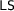constitute the scheduler models, which control the execution models by using a set of channels as scheduling commands. The execution models consist of two TA templates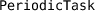anddescribing two types of tasks. We present the modeling methods of two major features of partitioned scheduling systemsFootnote 1.

Two-level Hierarchical Scheduling: The two-level scheduler modelsandrealize the hierarchical architecture. Take the local schedulershown in Fig.5 for example. A local scheduler belongs to a partition identified by a template parameter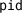.receives notification fromthrough two channels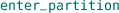and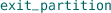when entering and exiting the partitionrespectively, and uses four channels,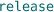,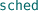andas commands to manage the tasks in. If there is a task becoming ready to run or relinquishing the processor, the task model will send itsaorcommand respectively.maintains a ready queue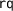that keeps all the tasks ready and waiting to run, and always allocates the processor to the first task with the highest priority in. If a new task having a higher priority than any tasks inget ready,will insert the task into, interrupt the currently running task viaand schedule the new selected task via.

According to whether the current time is inside the partition as well as to the number of the tasks in the ready queue, we create four major locations,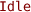,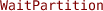, and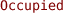. These four locations cover all situations, where the model must be at one of these locations for any instant. By contrast, the other locations realize conditional branches and atomic action sequences in the model.

Note that this framework has the capability of adopting different local scheduling policies in the system. This can be achieved by instantiating a new template of the local scheduler with a different scheduling policy for the partition. The new template is only required to conform with the same function definition of the channels as before.

Task Behavior In the templatesand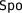-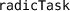, we define a set of abstract instructions to describe concrete task behavior. Figure 6 shows the main structure of the task templates. A clock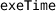measures the processing time during the execution of an abstract instruction, and progresses only when the model is at the location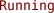. Once the task is scheduled bythrough the channel, it will start execution on the processor and move from the location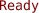to.

A sequential list of abstract instructions is implemented as the structure array. By using an integer variable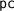as a program counter, the task can fetch the next abstract instruction from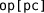at the location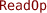. According to the command of this abstract instruction, the task model performs a conditional branch and moves from the locationto one of the different locations that represent different operations.

## 5 Evolutionary algorithm EA4HS

Evolutionary algorithms (EA) are an iterative stochastic search method inspired by natural selection and based on the collective learning process within a population of individuals, each of which represents a search point in the solution space of a specific problem . The population evolves from random initial values toward increasingly better solutions by means of three selection, recombination, and mutation operators. The individuals are evaluated and selected according to the value of a fitness function. There are several variants of EAs such as Genetic Algorithms (GA), Evolution Strategies (ES), and Evolutionary Programming (EP), which adopt distinctive fitness function, representation of search points, and implementation of operators.

In this section, we present an evolutionary algorithm EA4HS for solving the parameter optimization of ARINC-653 hierarchical scheduling systems. This algorithm combines improved operators of the GA and self-adaptation of the ES. We first give the outline of EA4HS. The designs of its fitness function, operators and self-adaptation are then detailed.

### 5.1 Outline of the evolutionary algorithm EA4HS

The goal of EA4HS is to optimize a set of object parameters $$\mathbf {x}=(x_1,x_2,\dots ,x_m)$$, i.e., the unknown 2n-dimensional vector $$\mathbf {x}$$ in the optimization problem, regarding an objective function $$\varOmega :\mathbb {R}^m_+\rightarrow \mathbb {R}$$. The EA manipulates populations $$\beta ^{(g)},g\in \mathbb {N}$$ of individuals $$\alpha ^{(g)}_k,$$ $$k=1,2,\dots ,K$$ where g is the number of generations and K the size of the population. An individual $$\alpha ^{(g)}_k$$ is represented by a tuple $$\langle \mathbf {x}^{(g)}_k,\mathbf {s}^{(g)}_k\rangle$$ that consists of not only object parameters $$\mathbf {x}^{(g)}_k=(x^{(g)}_{k,1},x^{(g)}_{k,2},\dots ,x^{(g)}_{k,m})$$ but also strategy parameters $$\mathbf {s}^{(g)}_k=(\sigma ^{(g)}_{k,1},\sigma ^{(g)}_{k,2},\dots ,\sigma ^{(g)}_{k,m})$$.

The strategy parameters come from evolution strategies to control statistical properties of the genetic operators . These strategy parameters can evolve together with object parameters during the evolution process. For any individual $$\alpha ^{(g)}_k$$, there are 2n strategy parameters in $$\mathbf {s}^{(g)}_k$$ where the evolution of $$x^{(g)}_{2i-1,k}$$ and $$x^{(g)}_{2i,k}$$ with $$i\in \{1,2,\dots ,n\}$$ (i.e. the unknown parameters $$p_i$$ and $$b_i$$ in the optimization problem) is guided by the combination of $$\sigma ^{(g)}_{2i-1,k}$$ and $$\sigma ^{(g)}_{2i,k}$$.

Let I be the range of individuals. The fitness function $$f:I\rightarrow \mathbb {R}$$ realizes the objective function $$\varOmega$$ by mapping each individual to a fitness value. In general, the better an individual fits, the higher is the probability of its being selected in the next generation. Moreover, the EA adopts the mechanism of elitism that many of the fittest individuals are copied directly to the next generation, and E is the number of elitist individuals in each generation.

The outline of EA4HS is given in Algorithm 2.

The object parameters in the first population $$\beta ^{(0)}$$ are initialized as a set of independent random numbers from a uniform distribution $$\mathcal {U}(x_{imin},x_{imax})$$ where the interval $$[x_{imin},x_{imax}]$$ indicates the search range of the optimal solutions. By contrast, all the strategy parameters are set to user-defined values at the first generation according to the definition of the mutation operator of object parameters. Then we evaluate the fitness value of each individual in $$\beta ^{(0)}$$ (line 3). After initialization we enter and execute the main loop of the evolution process until a termination condition is satisfied (lines 4–19).

The main loop produces a descendant population $$\beta ^{(g+1)}$$ from the parent population $$\beta ^{(g)}$$ at any generation g. First, E elitist individuals are copied into the set e (line 5). According to the fitness values of $$\beta ^{(g)}$$, we execute the selection operator that chooses $$(K-E)$$ pairs of parents separately from the population $$\beta ^{(g)}$$ and writes these parental individuals into the set $$\beta ^{(g)\prime }$$ (line 6). Then, the algorithm enters an inner loop (lines 7-14) where a new individual is born during each iteration.

In this inner loop, reproduction should be repeated until a valid object parameter combination is produced or the maximum number $$R_{ max }$$ of iterations is reached (lines 8-13). Otherwise the new generation would be drowning in invalid parameters and starved of information. Based on the selected parental pairs in $$\beta ^{(g)\prime }$$, the recombination and mutation of object parameters are performed (lines 10 and 12), generating the object parameter vector $$\mathbf {x}^{(g+1)}_k$$ of the kth new offspring. Meanwhile, the strategy parameters originating from $$\beta ^{(g)}$$ also undergo recombination (line 9) and mutation (line 11) independently to control the mutation operator of object parameters that achieves mutative self-adaptation. The resulting object parameters $$\mathbf {x}^{(g+1)}_k$$ and strategy parameters $$\mathbf {s}^{(g+1)}_k$$ constitute a new individual $$\alpha ^{(g+1)}_k$$.

We obtain the descendant population $$\beta ^{(g+1)}$$ by composing E elitist individuals e and $$(K-E)$$ new offspring $$\{\alpha ^{(g+1)}_k\}$$ (line 15). The fitness of $$\beta ^{(g+1)}$$ is evaluated (line 16) to update the current optimal scheduling parameters $$\mathbf {x}$$ (line 17). Finally, the evolution process returns $$\mathbf {x}$$ as an optimal solution (line 20).

### 5.2 Definition of the fitness function

The fitness function provides a measure for any individual $$\alpha =\langle \mathbf {x},\mathbf {s}\rangle$$ to determine which individuals should have a higher probability of being selected to produce the population at next generation.

The motivation for designing this fitness function stems from two aspects: First, the fitness value should reflect not only the goal of processor occupancy but also the potential for schedulability satisfaction. Such a fitness function evaluates the processor occupancy on the basis of assessment of the schedulability constraints in such a way that we select better individuals without breaching the constraints of the optimization problem. Second, it is necessary to speed up the fitness calculation due to a costly model-based schedulability analysis. An integration of global SMC testing and compositional MC verification should provide a fast strict assessment of schedulability properties for any individual.

Accordingly, the fitness function $$f:I\rightarrow \mathbb {R}$$ extracts the object parameters $$\mathbf {x}=(x_1,x_2,\dots ,x_{2n})$$ from their individual $$\alpha$$ and evaluates the fitness value of $$\mathbf {x}$$ in accordance with the following principles:

• Invalid parameter combinations, which cannot generate a valid partition schedule, are assigned to the lowest fitness.

• For any valid parameter vector $$\mathbf {x}$$, the MC verification should not be invoked to confirm the strict schedulability until the entire system of $$\mathbf {x}$$ is proved statistically schedulable by the SMC tests.

• Higher fitness values should be assigned to statistically schedulable parameter vectors than non-schedulable ones, and to strictly schedulable parameter vectors than only statistically schedulable ones.

• For any valid parameter vector $$\mathbf {x}$$, if more schedulable partitions are found in the SMC tests or MC verification, a higher fitness should be assigned to $$\mathbf {x}$$.

• For any two valid parameter vectors, if they are equal in the number of schedulable partitions, we will assign a higher fitness to the vector whose schedulable partitions occupy less processor time.

• For any strictly schedulable parameter vector, a lower processor occupancy U means a higher fitness.

We define the fitness function as the following piecewise formula:

\begin{aligned} f(\alpha ) = \left\{ \begin{array}{ll} -\zeta \Big (1+\frac{\sum _{i=1}^ng(x_{2i-1},x_{2i})}{\sum _{i=1}^nx_{2i}}\Big ), &{}\ \gamma _1\\ -\zeta \Big (1-\frac{1}{\sum _{i=1}^n(x_{2i}/x_{2i-1})}\Big ), &{}\ \gamma _2\\ 0, &{}\ \gamma _3\\ \zeta \Big (1+\sum _{i=1}^n\rho (i)(1-\frac{x_{2i}}{x_{2i-1}})\Big ), &{}\ \gamma _4\\ \zeta \Big (n+\frac{1}{n}\big (1+\sum _{i=1}^n\varphi (i)(1-\frac{x_{2i}}{x_{2i-1}})\big )\Big ), &{}\ \gamma _5\\ \zeta \Big (1+n+U_r(\mathbf {x})\Big ), &{}\ \gamma _6 \end{array} \right. \end{aligned}
(6)

where the conditions consist of

• $$\gamma _1:\ \exists i, x_{2i-1}<x_{2i}$$

• $$\gamma _2:\ \lnot \gamma _1\wedge \sum _{i=1}^n\frac{x_{2i}}{x_{2i-1}}>1$$

• $$\gamma _3:\ \lnot \gamma _1\wedge \lnot \gamma _2\wedge \lnot valid(\mathbf {x})$$

• $$\gamma _4:\ valid(\mathbf {x})\wedge \sum _{i=1}^n\rho (i)<n$$

• $$\gamma _5:\ valid(\mathbf {x})\wedge \sum _{i=1}^n\rho (i)=n\wedge \sum _{i=1}^n\varphi (i)<n$$

• $$\gamma _6:\ valid(\mathbf {x})\wedge \sum _{i=1}^n\rho (i)=n\wedge \sum _{i=1}^n\varphi (i)=n,$$

$$\zeta$$ is a scale factor, $$g(p,b) = \left\{ \begin{array}{ll}b-p, &{}\ p<d \\ 0, &{}\ p\ge d\end{array} \right.$$ provides the excess budget for the period p and execution budget b, $$\rho (i) = \left\{ \begin{array}{ll}1, &{}\ \text {if SMC query }\rho _i\text { is satisfied}\\ 0, &{}\ \text {if SMC query }\rho _i\text { is not satisfied}\end{array} \right.$$ returns the results of the SMC schedulability testing, Similarly $$\varphi (i) = \left\{ \begin{array}{ll}1, &{}\ \text {if TCTL property }\varphi _i\text { is satisfied}\\ 0, &{}\ \text {if TCTL property }\varphi _i \text { is not satisfied}\end{array} \right.$$ provides the results of the compositional MC schedulability verification, $$U_r(\mathbf {x})$$ gives the remaining processor utilization, and $$valid(\mathbf {x})$$ fetches the validity of $$\mathbf {x}$$ after invoking Algorithm 1. The condition $$\sum _{i=1}^n\rho (i)=n$$ and $$\sum _{i=1}^n\varphi (i)=n$$ imply the statistically and strictly schedulability, respectively, for all n partitions of the system conclude with positive results.

There are six cases in the definition of our fitness function. As shown in Fig.7, we allocate different ranges on the number axis to these cases.

The first three cases handle invalid parameter combinations that are indicated by negative or zero fitness values. In the first case $$\gamma _1$$, there exists a partition $$\mathcal {P}_i$$ whose execution budget $$b_i=x_{2i}$$ is greater than its period $$p_i=x_{2i-1}$$. Obviously, such a combination does not make sense. Thus, we compute the normalized sum of all the excess budgets and shift it to a low interval $$[-2\zeta ,-\zeta )$$. The second case $$\gamma _2$$, where the total utilization ratio $$\sum _{i=1}^nx_{2i}/x_{2i-1}$$ is greater than 1, overspends all available budgets. Similarly, the excess ratio is mapped into the interval $$(-\zeta ,0)$$. The rest of invalid parameter vectors should be reported by the ARINC-653 schedule generator due to the non-schedulability at the global level. They are classified as the third case $$\gamma _3$$ and assigned zero fitness. Note that the model-based schedulability testing or verification is not required in these invalid cases.

On the contrary, the fitness of valid object parameters is evaluated on the basis of the results of SMC tests and MC verification. After fast testing the schedulability of each partition inSMC, we calculate a fitness value according to the number of statistically schedulable partitions $$n_s=\sum _{i=1}^n\rho (i)$$. The fitness value is mapped into the interval $$[n_s\zeta ,(n_s+1)\zeta )$$ by adding $$\zeta n_s$$ and the normalized remaining utilization ratio of statistically schedulable partitions $$\zeta (1-\sum _{i=1}^n\rho (i)x_{2i}/x_{2i-1})$$ (i.e. case $$\gamma _4$$).

Not until all n partitions go through the SMC tests will the costly compositional MC method be invoked to verify the schedulability of the system. Once this property is confirmed (i.e., case $$\gamma _6$$), the fitness function will extend the remaining processor utilization $$U_r$$ by an offset $$(n+1)\zeta$$, thus obtaining the highest fitness within $$[(n+1)\zeta ,(n+2)\zeta )$$. If the schedulability of the system is falsified by the MC verification (i.e. case $$\gamma _5$$), we will map the sum of the number of strict schedulable partitions $$\sum _{i=1}^n\varphi (i)$$ and their remaining utilization ratio $$(1-\sum _{i=1}^n\varphi (i)x_{2i}/x_{2i-1})$$ into the interval $$[n\zeta ,(n+1)\zeta )$$.

### 5.3 Selection operator

In the evolution process, there is a high probability of producing low-fitness object parameters such as the invalid combinations where an execution budget is greater than its partition period. Since each generation contains many bad and only very few good individuals, we prefer exponential ranking selection operator that is able to give higher selective pressure, i.e., the tendency to select better individuals from a population , while guaranteeing certain standard deviation of the fitness distribution of the population after a selection operation .

Exponential ranking selection is implemented as two steps: (1) K individuals in a population are ranked in order of fitness from worst 1 to best K. (2) The ith individual is selected according to the exponentially weighted probability

\begin{aligned} p_i=\frac{c^{K-i}}{\sum _{j=1}^{K}c^{K-j}} \end{aligned}
(7)

where the base of exponent $$c\in (0,1)$$ is used to control the selective pressure of the operator. A smaller c will lead to a higher selective pressure, which means best-fitness individuals are more likely to be selected. The selection operation is repeated until $$(K-E)$$ pairs of individuals are obtained.

### 5.4 Recombination operator

There is a widely accepted design principle that recombination operators mainly extract the similarities from selected parents . In our optimization problem, the similarities between individuals originate not only from the independent values of partition periods and budgets but from the processor usage of each partition. Accordingly, we design a local line recombination operator for the EA4HS. For any partition $$\mathcal {P}_i$$, the recombination operator mixes information from parents about the period $$p_i$$ and budget $$b_i$$ of $$\mathcal {P}_i$$, and extracts the similarities in terms of the utilization ratio of $$b_i$$ to $$p_i$$, which indicates the processor usage of $$\mathcal {P}_i$$.

Let $$\mathbf {x}=(x_1,x_2,\dots ,x_m)$$ and $$\mathbf {y}=(y_1,y_2,\dots ,y_m)$$ be the object parameters of two parents. The local line recombination computes an offspring $$\mathbf {z}=(z_1,z_2,\dots ,z_m)$$ by

\begin{aligned} z_j=x_j+\xi _i(y_j-x_j)\quad j=1,2,\dots ,m\quad i=\left\lfloor \frac{j+1}{2}\right\rfloor \end{aligned}
(8)

where the weighting $$\xi _i$$ is randomly generated by a uniform distribution $$\mathcal {U}(-d,1+d)$$ and $$d\in [0.25,0.5]$$ is the constraint value on the line extension. For any offspring $$\mathbf {z}$$, two consecutive parameters $$p_i=z_{2i-1}$$ and $$b_i=z_{2i}$$ belonging to one partition share a common factor $$\xi _i$$. In doing so, the recombination produces the offspring parameters of each partition independently on a common line segment through both of the parents.

We consider three types of genetic information: (1) the period $$p_i$$, (2) the budget $$b_i$$, and (3) the utilization ratio $$b_i/p_i$$ of the ith partition. As depicted in Fig.8, the offspring $$\mathbf {z}$$ can be chosen uniformly at random from the line $$\mathbf {xy}$$, where the recombination operator mixes these three types of genetic information simultaneously from parents. Obviously, all three types of genetic information are kept in the offspring $$\mathbf {z}$$ and similar to those in its parents $$\mathbf {x}$$ and $$\mathbf {y}$$.

### 5.5 Mutation operator

Compared with recombination, mutation operators do not only provide a source of genetic variation but also maintain degree of population diversity, whose insufficiency is one of the major cause of premature convergence . However, generic mutation operators cannot utilize the correlations between the period $$p_i$$ and budget $$b_i$$ in individuals to acquire promising processor usage of partitions, causing the mutants to be always eliminated after selection in all probability. This extremely low survival rate increases the risk of premature convergence. Thus, we propose a rotated Gaussian mutation operator to help the EA4HS converge to a global optimum effectively.

The mutation operator has two input parameters including the set of object parameters $$\mathbf {z}=(z_1,z_2,\dots ,z_m)$$ after recombination and of strategy parameters $$\mathbf {s}=(\sigma _1,\sigma _2,\dots ,\sigma _m)$$ that control mutation strength. Each pair of the object parameters $$(z_{2i-1},z_{2i})$$ is mutated as an independent vector $$\tilde{z}_i$$. The mutation operator transforms $$\mathbf {z}$$ into a new offspring $$\mathbf {z}^\prime =(z^\prime _1,z^\prime _2,\dots ,z^\prime _m)$$. Let $$\tilde{z}^\prime _i$$ stand for $$(z^\prime _{2i-1},z^\prime _{2i})$$. We have

\begin{aligned} \tilde{z}^\prime _i=\tilde{z}_i+\varDelta _i\quad i=1,2,\dots ,n \end{aligned}
(9)

where $$\varDelta _i$$ is a random sample from a bivariate normal distribution $$\mathcal {N}(\varvec{\mu }_i,\varvec{\Sigma }_i)$$.

The covariance matrix $$\varvec{\Sigma }_i\in \mathbb {R}^{2\times 2}$$ can be geometrically interpreted as a set of ellipses, each of which is a density contour of $$\mathcal {N}(\varvec{\mu }_i,\varvec{\Sigma }_i)$$. Consider the fact that a parent $$\mathbf {z}$$ with valid parameters has a high probability of producing a valid offspring $$\mathbf {z}^\prime$$ if each of the new utilization ratios $$z^\prime _{2i}/z^\prime _{2i-1}$$ is close to the parental $$z_{2i}/z_{2i-1}$$. We define the covariance matrix $$\varvec{\Sigma }_i$$ as the set of ellipses whose major axes are parallel with the lines of equal ratio $$z_{2i}/z_{2i-1}$$ shown in Fig.8. Thus, $$\mathcal {N}(\varvec{\mu }_i,\varvec{\Sigma }_i)$$ is obtained by rotating a bivariate normal distribution $$\mathcal {N}(\varvec{\mu }_i,\mathbf {D}_i)$$ counterclockwise through an angle $$\theta _i$$:

\begin{aligned} \varvec{\Sigma }_i=\mathbf {R}_i\mathbf {D}_i\mathbf {R}_i^T \end{aligned}
(10)

where $$\mathbf {D}_i=diag(\sigma _{2i-1},\sigma _{2i})$$ derives two strategy parameters $$\sigma _{2i-1}$$ and $$\sigma _{2i}$$ from $$\mathbf {s}$$, $$\mathbf {R}_i=\left( \begin{array}{cc} cos\theta _i &{} -sin\theta _i \\ sin\theta _i &{} cos\theta _i \end{array} \right)$$ is a rotation matrix, and

\begin{aligned} sin\theta _i=\frac{z_{2i}}{\sqrt{z^2_{2i-1}+z^2_{2i}}},\ cos\theta _i=\frac{z_{2i-1}}{\sqrt{z^2_{2i-1}+z^2_{2i}}}. \end{aligned}
(11)

Strategy parameters $$\sigma _{2i-1}$$ and $$\sigma _{2i}$$ indicate the standard deviations of $$\mathcal {N}(\varvec{\mu }_i,\varvec{\Sigma }_i)$$ along the major and minor axes, respectively. To perform such an ellipses-parallel mutation, we initialize each $$\sigma _{2i-1}$$ of the strategy parameters with a greater value than $$\sigma _{2i}$$, thereby adapting the mutation distribution $$\varDelta _i\sim \mathcal {N}(\varvec{\mu }_i,\varvec{\Sigma }_i)$$ to the fitness landscape.

The mean $$\varvec{\mu }_i\in \mathbb {R}^2$$ of the normal distribution is defined as

\begin{aligned} \varvec{\mu }_i= \left\{ \begin{array}{ll} \mathbf {0}, &{}\ \Vert \tilde{z}_i\Vert _2\ge 2\sigma _{2i-1}\\ 2\sigma _{2i-1}(cos\theta _i,sin\theta _i), &{}\ \Vert \tilde{z}_i\Vert _2<2\sigma _{2i-1} \end{array} \right. \end{aligned}
(12)

where $$\Vert \tilde{z}_i\Vert _2=\sqrt{z^2_{2i-1}+z^2_{2i}}$$ is the Euclidean norm of the vector $$\tilde{z}_i$$. In most cases, the mean $$\varvec{\mu }_i$$ is assigned $$\mathbf {0}$$ and hence the mutants $$\mathbf {z}^\prime$$ will center around the input parameters $$\mathbf {z}$$. However, the zero mean $$\varvec{\mu }_i=\mathbf {0}$$ may cause the mutations to generate a large number of invalid minus parameters, especially when the input points $$\tilde{z}_i$$ are close to the origin but their mutations receive large standard deviations $$\sigma _{2i-1}$$. According to the empirical rule in statistics (i.e., 95% of the values in a normal distribution lie within two standard deviations of the mean), we will add a $$2\sigma _{2i-1}$$ offset along the major axis of $$\mathcal {N}(\mathbf {0},\varvec{\Sigma }_i)$$ to $$\tilde{z}_i$$ if the Euclidean norm of $$\tilde{z}_i$$ is less than a distance of $$2\sigma _{2i-1}$$, effectively reducing the probability of producing minus parameters.

Subsequently, the EA4HS sets strategy parameters adaptively to direct the search during the evolution process.

### 5.6 Self-adaptation of strategy parameters

The strategy parameters are encoded, selected and inherited together with the object parameters of individuals. They also undergo recombination and mutation operations to control the statistical properties of the mutation operator of object parameters adaptively.

Since the considerable fluctuations of strategy parameters normally degrade the performance of EAs , we provide a weighted intermediate recombination operator for strategy parameters in order to mitigate these fluctuations as well as extract the similarities. The recombinant $$\bar{\mathbf {s}}$$ is a weighted average of all the K vectors $$\mathbf {s}^{(g)}_k=(\sigma ^{(g)}_{k,1},\sigma ^{(g)}_{k,2},\dots ,\sigma ^{(g)}_{k,m})$$ of the strategy parameters in a population $$\beta ^{(g)}=\left\{ \alpha ^{(g)}_1,\alpha ^{(g)}_2,\dots ,\alpha ^{(g)}_K\right\}$$:

\begin{aligned} \bar{\mathbf {s}}=\frac{1}{K}\sum _{k=1}^K(1-\tau _r)^{\lambda _k}\mathbf {s}^{(g)}_k \end{aligned}
(13)

where $$\tau _r\in [0,1]$$ is a user-defined learning rate and $$\lambda _k$$ is the number of times an individual $$\alpha ^{(g)}_k$$ appears continuously in the elitist set $$\varepsilon$$. The recombination also assigns $$\bar{\mathbf {s}}$$ to all the individuals in $$\varepsilon$$.

A log-normal operator  is applied to the mutation of strategy parameters, providing the primary source of their genetic variation. This log-normal mutation ensures positiveness of the strategy parameters that serve as standard deviation of a normal distribution. The recombinant $$\bar{\mathbf {s}}=(\bar{\sigma }_1,\bar{\sigma }_2,\dots ,\bar{\sigma }_m)$$ mutates into the strategy parameters $$\mathbf {s}^{(g+1)}_k=(\sigma ^{(g+1)}_{k,1},\sigma ^{(g+1)}_{k,2},\dots ,\sigma ^{(g+1)}_{k,m})$$ at next generation by

\begin{aligned} \sigma ^{(g+1)}_{k,j}=\bar{\sigma }_je^{\tau _u\mathcal {N}_j(0,1)} \end{aligned}
(14)

where $$\tau _u$$ is also an input learning rate and $$\mathcal {N}_j(0,1)$$ denotes a random sample from the standard normal distribution. The learning rate $$\tau _u=1/\sqrt{m}$$ is recommended according to .

## 6 Experiments

This section presents the experiments on two avionics systems to demonstrate the applicability of our optimization method. In the experiments, our parameter sweep method shows the capability of converging to a global optimum. We also evaluate the performance of search algorithms by comparing the proposed EA4HS with exhaustive search and two popular genetic algorithms.

All the experiments in this section were executed on the cluster that consists of four computer nodes with 1 TB memory. Each node has 64 cores of 4 AMD Opteron 6376 processors. The schedulability tests and validation were performed on4.1.19 64-bit version. We assign the timebound $$N=1.0\times 10^4$$ time units and the probability threshold $$\theta =0.05$$ for Eq.(2).

### 6.1 Experiment on simple periodic task sets

We first perform the experiments on a simple periodic task set taken from . The task set comprises two identical partitions with different priorities, thus making their partition priority ordering irrelevant. Each partition contains multiple independent periodic tasks, whose period, deadline and priority are encoded intodeclarations. The behavior of a task is described as a pure $$Compute$$ instruction with a Worst Case Execution Time (WCET).

The task set is shown in Table 1 where the column “PID” and “TID” identify the partitions and tasks, respectively, “PR” gives partition priorities, “T” is task periods, “E” is the WCET, “D” is deadline, and “R” is task priorities. We define the time unit as a microsecond in the table and the context switch overhead as 2 time units.

#### 6.1.1 Experiment 1

The parameter optimization of the above avionics workload was carried out by three following methods:

• Exhaustive search An analytical condition for schedulable parameters is derived from a response time analysis . This method scans all possible integer combinations of the partition period through a potential interval [4, 200]. For each period combination, it uses a binary search together with the schedulability condition to find the minimum execution budget from the highest priority to the lowest one. This exhaustive search is able to produce a global optimal solution, but only applicable to such a simple system.

• Parameter sweep with GAs Two popular GAs, the classic and the breeder genetic algorithm , are first applied to parameter sweep for comparison. Table 2 shows their operator combinations and denotes them by “GA 1” and “GA 2” respectively. Individuals are binary encoded in both of the GAs. In GA 1, an exchange of each bit in parents takes place with a probability $$p_e=0.5$$, and the probability of bit mutation is $$p_u=0.2$$. In GA 2, the percentage $$T\%$$ of truncation selection is set to $$50\%$$, the weighting constraint of intermediate recombination is $$d_r=0.5$$, the standard deviation of Gaussian mutation is $$\sigma _u=10$$, and the mutation probability is $$p_u=0.2$$.

• Parameter sweep with EA4HS In the EA4HS, individuals are also binary encoded, the base c of exponential ranking selection is 0.8, the value d of local line recombination is 0.5, and we initialize the strategy parameters $$\sigma _{2i-1}=50$$ and $$\sigma _{2i}=5$$ for $$i\in \{1,2\}$$ in an individual. We define the learning rates $$\tau _r=0.7$$ and $$\tau _u=1/\sqrt{4}=0.5$$. The operator combination is also shown in Table 2.

Both the GAs and EA4HS adopt the search range [4, 200] for all partition periods, population size $$K=64$$, elitism size $$E=4$$, and maximum generation $$G=300$$. For each individual, we calculate its fitness value and store them in a hash table. Once the same individual reappears in the following generations, the fitness value will be fetched from the hash table directly, thus avoiding the costly redundant fitness calculation.

Table 3 shows the optimization result of Experiment 1. Both the exhaustive search and parameter sweep with the EA4HS reached the same global optimal solution $$\mathbf {x}_{opt}=(160,34,160,34)$$, which gives a minimum processor occupancy $$U=45\%$$. Unfortunately, two GAs only offer two local optimal solutions with much higher processor occupancy $$61.67\%$$ and $$58.73\%$$.

Figure 9 presents the evolution of minimum processor occupancy and cumulative processing time of the GAs and EA4HS in Experiment 1. Since duplicate fitness calculation is replaced with reading the hash table, the convergence of the evolution means a synchronous slowdown in the variation of minimum processor occupancy and cumulative processing time. Obviously, both GA 1 and GA 2 fell into a premature convergence on local optimal solutions after 30 generations.

In contrast, the EA4HS adjusts strategy parameters adaptively to control the average search area of mutation operations. When there was a convergence trend during the generations of [30, 70) and [160, 200), the self-adaptation of strategy parameters expanded the search areas to improve population diversity and subsequently made the search concentrated in smaller areas to find better solutions within few generations, thereby leading to a fast decrease in the minimum processor occupancy over the subsequent generations [100, 160) and [270, 300) shown in Fig. 9a. The repeated adjustments of the EA4HS reduce the risk of premature convergence on a local optimal area, producing two “steps” of its processing time curve in Fig. 9b.

#### 6.1.2 Experiment 2

Considering that the premature convergence may affect the result of these two GAs, we continue the comparison experiment on the same task set but adopt different configuration for the EAs to defer their convergence during the evolution. Experiment 2 repeats the same procedure for Experiment 1, using the same search range [4, 200], population size $$K=64$$, elitism size $$E=4$$, maximum generation $$G=300$$, and the following detailed configuration:

• GA 1 More frequent bit-flip mutation in GA 1 will produce new individuals more randomly, thus possibly raising the degree of population diversity to prevent premature convergence. Hence we keep the bit-exchange probability $$p_e=0.5$$ but use a double bit-mutation probability $$p_u=0.4$$.

• GA 2 We increase both the probability and strength of the variable mutation to delay the convergence in GA 2, using the new standard deviation $$\sigma _u=20$$ of Gaussian mutation and its larger mutation probability $$p_u=0.4$$. We still keep the percentage $$T=50\%$$ of truncation selection and the weighting constraint $$d_r=0.5$$ of intermediate recombination.

• EA4HS In the EA4HS, a lower learning rate $$\tau _r$$ will slow down the convergence by adjusting the average strategy parameters of populations. Hence we invoke the EA4HS with a smaller learning rate $$\tau _r=0.4$$ and retain the other configuration including the base $$c=0.8$$ of exponential ranking selection, the weighting constraint $$d=0.5$$ of local line recombination, the learning rate $$\tau _u=0.5$$, and the initial strategy parameters $$\sigma _{2i-1}=50$$ and $$\sigma _{2i}=5,i\in \{1,2\}$$.

In Table 4, the results of Experiment 2 show that our EA4HS gets accustomed to this new configuration, for the algorithm acquired the global optimal solution $$\mathbf {x}_{opt}=(160,34,160,34)$$ with the minimum processor occupancy $$U=45\%$$. Unfortunately, two GAs still deviated from the global optima, but they generated better solutions compared with their optimization result in Experiment 1.

Figure 10 depicts the evolution of minimum processor occupancy and cumulative processing time in Experiment 2. Although GA 1 obtained a lower processor occupancy than that in Experiment 1, it fell into the premature convergence again at around the 30th generation. The new configuration of GA 2 successfully avoided its convergence to find more better individuals but significantly increased the processing time from 347 to 2833 min.

Compared with the configuration of EA4HS in Experiment 1, a lower learning rate $$\tau _r=0.4$$ avoids a sharp drop in strategy parameters and frequent adjustments during the evolution. Thus the EA4HS generated smoother curves of minimum processor occupancy (Fig. 10a) and cumulative processing time (Fig. 10b) in Experiment 2. The evolution had not entered a convergence until it found a nearly optimal solution at around the 200th generation, finally reaching the global optimum at the 212nd generation.

The experiments reveal distinct superiority of our EA4HS over the GAs. First, GA 1 is not applicable to this optimization problem. We find the bit-based reproduction of GA 1 cannot produce more better individuals steadily, making GA 1 very prone to premature convergence. By contrast, GA 2 can overcome the problem of premature convergence by adjusting the mutation configuration. However, GA 2 has a low search efficiency. In both of the experiments, GA 2 concentrate search on the local optimal area where all the periods are centered around $$120~\upmu \text {s}$$ and far from the best $$160~\upmu \text {s}$$. For this purpose, our EA4HS is provided with new recombination and mutation operator which can produce descendant individuals on the basis of the processor usage of parents. Hence, it is more likely to climb up a higher processor occupancy even if our current individuals are far from the optimal area.

### 6.2 Experiment on a concrete avionics system

We undertake the third experiment on a much larger and more complex IMA partitioned scheduling system including multiple task types, task dependency, and inter-partition communication [11, 16]. As shown in Table 5, the system consists of five partitions that contain a total of 18 periodic tasks and four sporadic tasks. The type of a task depends on its release interval. A periodic task has a fixed period, whereas a sporadic task satisfies a minimum separation between consecutive release. The execution of a task is characterized as a sequence of chunks. Each chunk has a lower and upper bound on execution time, a set of potentially required resources and message-passing operations. There are 3 intra-partition locks, as shown in column mutex, and 4 inter-partition message types in the task set. The columns output and input indicate transfer direction of messages. According to the resources required by chunks, we convert each chunk into a subsequence of the abstraction instruction sequence $$( Receive,$$ $$Lock,$$ $$Compute,$$ $$Unlock,$$ $$Send,$$ $$End )$$ in theexecution models. We assume the context switch overhead to be 0.2 milliseconds in the experiment.

In this IMA system, the features such as task dependency and communication render the analytical bounds in  non-applicable. Moreover, the immense parameter space makes it impossible to complete a brute-force search for a global optimal solution. Hence, Experiment 3 compares the EA4HS optimization with the empirical scheduling scheme given in . Their detailed configuration is listed as follows:

• Empirical scheduling In this scheme, all the partitions have a unique period $$p=25~\text {ms}$$, which is the minimum and a harmonic of task periods. Each partition is allocated to a time slot of the same length $$5~\text {ms}$$ within every partition period. We first create an ARINC-653 partition schedule according to this empirical scheme. The common partition period is used as the major time frame $$M=25~\text {ms}$$. Within every M, the five time slots of the partitions are arranged in order of priority. A context switch overhead $$0.2~\text {ms}$$ is inserted into the start of the time slots, each of which thus shrinks to the size of 4.8 ms. Subsequently, we analyze the schedulability of this empirical scheme by using the compositional approach given in Sect. 3.

• EA4HS Considering the larger parameter space and longer processing time for each generation, we apply a new population size $$K=256$$, elitism size $$E=16$$ and maximum generation $$G=200$$ but keep the rest of the configuration of Experiment 2, which has been proved applicable to the simple system with similar quantities of time. We set the base of exponential ranking selection $$c=0.8$$ and the value of local line recombination $$d=0.5$$. In the first generation, the strategy parameters of an individual are initialized as $$\sigma _{2i-1}=50$$ and $$\sigma _{2i}=5$$ for $$i\in \{1,2,\dots ,5\}$$. We define the learning rates $$\tau _r=0.4$$ and $$\tau _u=1/\sqrt{10}\approx 0.3$$.

Table 6 presents the optimization results of Experiment 3. Owing to the much larger unknown parameter space, it is more difficult to find a schedulable solution than Experiment 1 and 2. The empirical scheme even failed to conclude with a schedulable solution. In the schedulability analysis, a counterexample generated bydemonstrates that $$Tsk ^{\textit{1}}_{\textit{3}}$$ misses its deadline at the instant $$t=50.2~\text {ms}$$. Although the original $$5~\text {ms}$$ allocation is sufficient for the execution of $$Tsk ^{\textit{1}}_{\textit{3}}$$, the additional overhead of context switches makes $$Tsk ^{\textit{1}}_{\textit{3}}$$ go over budget. Moreover, this empirical scheme takes up all the processor time, increasing the integration cost of additional avionics workload.

In contrast to the unsatisfactory results of the empirical method, our EA4HS acquired a schedulable solution $$\mathbf {x}=(25, 4.9, 25, 4.7, 25, 3.4, 25, 4.5, 50, 4.5)$$ with a lower processor occupancy $$82.6\%$$. Its schedulability is not only tested statistically bySMC but also validated rigorously byclassic MC. Even though its global optimality cannot be confirmed, engineers can still benefit from such schedulable results that have acceptable processor occupancy.

Figure 11a illustrates the best fitness value and processing time of each generation in the EA4HS optimization. The fitness value offers the quality evaluation of any parameter combination regardless of its schedulability. According to the definition of fitness function in Sect. 5.2, the coordinate plane can be divided into three areas that correspond to different fitness intervals: (1) [0, 500) where the generations contain no schedulable solution, for all the individuals are fast falsified by SMC. (2) [500, 600) where all five partitions of the best individual are proved statistically schedulable by SMC but its schedulability is finally excluded by MC. (3) [600, 700) where the schedulability of the best individual is strictly confirmed by MC.

As shown in Fig. 11a, there was no schedulable individual in the initial population. During the generations of [1, 105), the best fitness value and processing time increased gradually as more partitions of individuals were proved statistically schedulable by SMC. At the 105th generation, we found the first statistically schedulable individual with the fitness value 501 and started the MC compositional analyses. Although its schedulability was excluded by MC, there were a growing number of higher-fitness individuals that went through the SMC tests at the following generations. Since most of the MC compositional analyses were much more time-consuming than the SMC tests, the average processing time for each generation rose from around 10min to more than 40min after 105 generations. Finally, we acquired the first schedulable individual at the 161st generation and found the best solution with the lowest processor occupancy $$82.6\%$$ at the 179th generation within the cumulative time of 62 hours.

Figure 11b shows the composition of populations during the evolution. A population consists of the following four types of individuals: (1) Invalid individuals that cannot generate ARINC-653 schedules. (2) SMC falsified individuals that turned out to be non-schedulable in the SMC tests. (3) MC falsified individuals that were proved statistically schedulable by SMC but eliminated in the MC compositional analyses. (4) schedulable individuals.

The evolution of the population composition demonstrates improvements in the efficiency of our EA4HS optimization. First, the EA4HS avoids the populations drowning in invalid individuals via repeated reproduction (lines 8–13 of Alg. 2). As shown in Fig. 11b, invalid individuals accounted for a quarter of the initial population that was generated randomly, but the EA4HS kept their proportion falling sharply until they vanished after the 17th generation. Second, the application of SMC fast falsification speeds up the optimization. For each population in Fig. 11b, most of the individuals underwent the SMC tests rather than the costly MC compositional analyses. Third, the EA4HS adaptively keeps a steady growth in the number of higher-fitness individuals but does not concentrate rapidly on the localities of dominant solutions, thus reducing the risk of premature convergence. In Fig. 11b, the proportion of schedulable individuals increased gradually until they filled the elitist list. At the following generations, the newly-produced individuals did not converge on a few dominant solutions but maintained a degree of population diversity. Finally, there was a steady proportion of schedulable individuals in the populations at around the 200th generation.

## 7 Related work

A few approaches to optimizing the partition scheduling of avionics systems have been presented in the literature, applying analytical or formal methods from either a global or compositional viewpoint on the hierarchical scheduling architecture.

Compositional analytical methods introducing the abstraction and composition of constituent partitions optimize each partition locally for the whole system. The authors of [15, 28, 29] adopted different resource models to characterize the time demand and supply of partitions, presented the schedulability conditions under EDF (Earliest Deadline First) and RM (Rate Monotonic) policy, and gave utilization bounds of these resource models. In , they extended this compositional framework into ARINC-653 avionics systems, providing a task model to deal with the behaviors like communication latencies and blocking/preemption overheads within partitions. In , the authors proposed a similar analytical method for applications consisting of periodic or sporadic tasks scheduled by FP policy to find the best scheduling parameter pairs of partitions. To improve the runtime performance, Dewan and Fisher  proposed a polynomial-time approximation algorithm for minimizing the interface bandwidth of sporadic task systems.

However, the combination of local optimality of each partition does not necessarily lead to the globally optimal solution, because the parameters chosen for one partition may affect the choice for other partitions . From a global viewpoint, Davis and Burns  formulated the optimization problem as a holistic selection of partition parameters, providing a set of search algorithms to find the best parameter combination. Nevertheless, the optimal solution can only be determined by an exhaustive search in the case of small systems. Yoon et al.  showed the non-convexity of multiple partition optimization and solved this nonlinear non-convex problem with Geometric Programming (GP). Kim et al.  formulated a linear programming problem for the utilization bound of a schedulable periodic task set scheduled by RM policy in a given ARINC-653 partition. Blikstad et al.  simplified the two-level hierarchical scheduling into the pre-runtime scheduling of non-overlapping periodic tasks, adopting a Mixed Integer Programming (MIP) formulation to generate the optimal schedule.

Unfortunately, both of the analytical methods introduce a certain degree of pessimism due to the oversimplification of their optimization policies or system models:

The pessimism of the compositional methods mainly originates from the oversimplification of optimization policies. The compositional methods employ the “Divide and Conquer” strategy, finding the optimal solution for each partition independently and assembling all the local results as a complete solution of the system. For each partition, such solvers always search for its optimal solution on the worst-case assumption of the rest of the partitions. This policy reduces the complexity of optimization solving but ignores the possible coordination between partitions. Hence the final solution of an ARINC-653 partitioned system is only the combination of local optima and not globally optimal.

The pessimism of the global optimization methods is introduced by the oversimplification of system models. Since the nature of ARINC-653 partition scheduling is a complex nonlinear non-convex optimization problem , these global methods simplify the system models by linearizing the analytical equations of the schedulability constraints and formulate it as a classical optimization problem like MIP. This simplification leads to a conservative solution of partition scheduling. The degree of pessimism depends on the approximation precision of the simplified system model.

By contrast, model-based methods provide rigorous formal models to describe more concrete behaviors of avionics systems in a readable and understandable way.

Beji et al.  expressed the constraints of distributed IMA architecture as SMT (Satisfiability Modulo Theory) logic formulas and used the SMT solver YICES to find automatically feasible scheduling parameters that minimize the integration cost. However, modeling a detailed system requires a large number of lengthy logic formulas. Their SMT model only covering a flat rather than hierarchical structured partition scheduling system did not give any specification of the tasks in partitions.

Sun et al.  proposed a component-based schedulability analysis of hierarchical scheduling systems encoded into linear hybrid automata, thus enabling the optimization of partition parameters. All the integer values of partition parameters were exhaustively tested for schedulability to minimize the processor utilization. Obviously, this exhaustive search is not feasible for a large high-dimensional parameter space.

The authors of [8, 22] appliedto the compositional optimization of partition parameters. Given a specific partition, they used a lightweight SMC method for a fast design exploration of objective parameters, assuring the schedulability of the corresponding TA models with a high confidence. Once a promising parameter tuple had been found, it could be proved schedulable using the costly MC method. This approach mitigating the state-space explosion coped with each partition up to 6 tasks. However, as remarked above, this compositional optimization does not necessarily lead to the globally optimal solution. Moreover, they also ignored concrete task behaviors in the TA models.

In summary, the analytical methods build on a rigorous mathematical deduction under the worst-case assumptions of a simplified system, thereby fast solving the optimization problem at a low cost. By contrast, the model-based methods are more expressive to describe a concrete avionics system but their optimizers face the challenge of the complexity problem. In this paper, our model-based approach adopts a global evolutionary search to explore the objective solution space effectively and uses the integrated method of simulation-based tests and compositional verification to make the costly schedulability analysis feasible.

## 8 Conclusion

The model-based method presented in this paper addresses the optimization problem of ARINC-653 partition scheduling in a complex IMA system. We conclude that our model-based approach is applicable to this optimization problem, where an IMA system is modeled as a network of timed automata in. Compared with widely-used analytic optimization, the timed automata model of our method is more expressive to describe complex features of IMA systems. We formulate the problem as a global search for the optimal partition scheduling parameters that achieve the minimum processor occupancy and meet the schedulability constraints. A parameter sweep optimizer explores the solution space via evolutionary algorithm while guaranteeing the schedulability by model checking. The evolutionary algorithm EA4HS is promising for reaching the optimal parameters quickly as well as avoids exhaustive exploration of the solution space. The combination of global SMC testing and compositional model-checking verification alleviates the state space explosion of classical model checking. The experiments demonstrate that our optimizer is able to identify the global optimum solutions for simple task sets and find acceptable ones effectively for complex systems.

The design of the model-based parameter sweep also introduces limitations into this optimization method. First, the evolutionary search may not reach a global optimal solution within a finite number of generations, especially when handling a complex IMA system with a large number of partitions. However, our method is still capable of producing better high-quality candidates than purely empirical scheduling schemes. Engineers can benefit from the optimization results of our method in integrating a set of complex applications. Second, the model-based method involves frequent time-consuming schedulability analyses, leading to a long processing time from a few hours to days. But we believe that such a processing time is negligible in the development life cycle of an IMA system. Moreover, we can speed up the optimization process by running on more powerful clusters. As future work, we plan to add more features such as multi-core processor support and more local scheduling policies to the system, further generalizing the proposed method to more complex ARINC-653 scheduling systems.